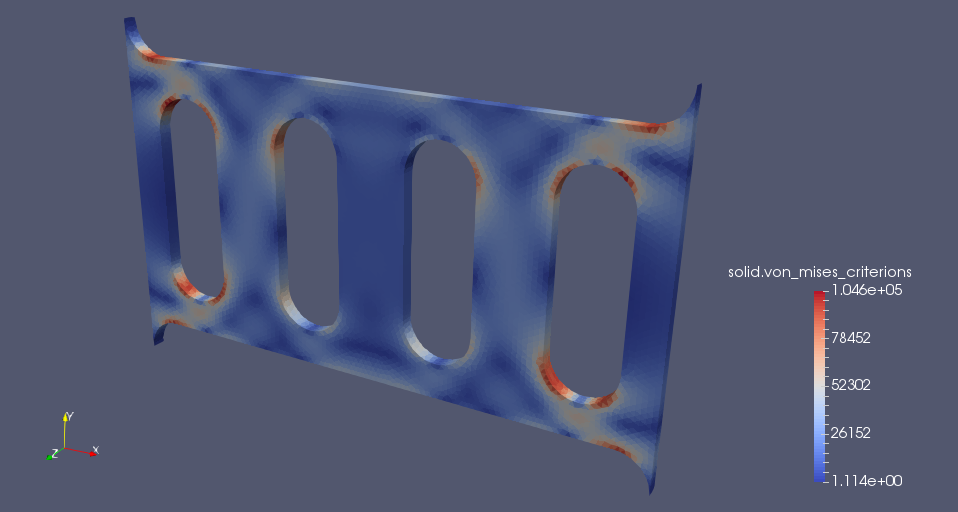# Vierendeel truss

## 1. Introduction

A Vierendeel truss is a lightweight deep beam or girder structural concept. The goal of this exercise is to determine the deformation and stress state of the aluminium Vierendeel truss shown below, in particular the maximum stress around the lightening holes.

## 2. Running the case

The command line to run this case is

``feelpp_toolbox_solid --config-file Plate.cfg``
 The report of the execution of the command above is available here.

## 3. Model/Geometry

First, we need to build the model, which we are going to do using the Gmsh-software.

The overall dimensions of the model are: 200x125x5 mm.

Dimensions in the Gmsh-model are in meters.

The finished geometry and the meshed model with 2 mm mesh-size: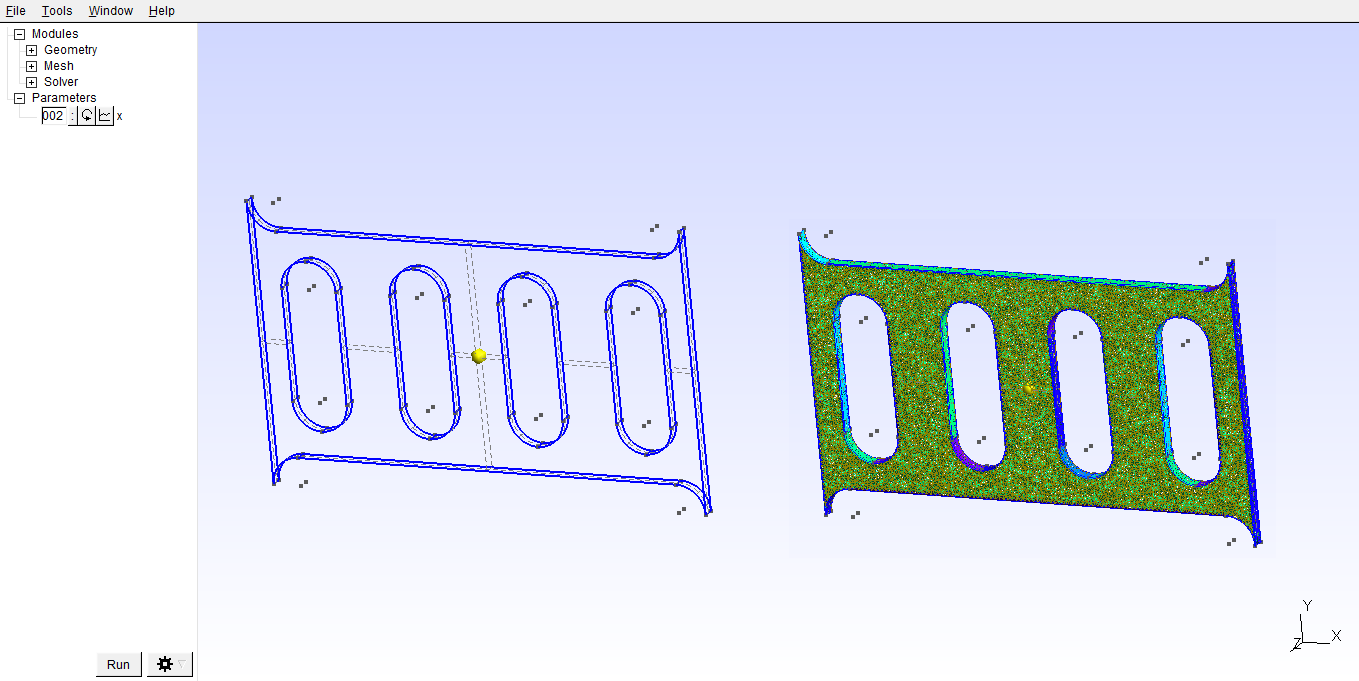## 4. Materials and boundary conditions

### 4.1. Materials

The beam is made of steel, so we need to use the following data:

• E = 69 GPa

• nu = 0.3

• rho = 2712 kg/m3

### 4.2. Boundary conditions

Both ends of the truss are fixed and the force is applied to the upper surface. In this case we will apply max. 10000 N force to the upper surface (named „force”) as a function of x.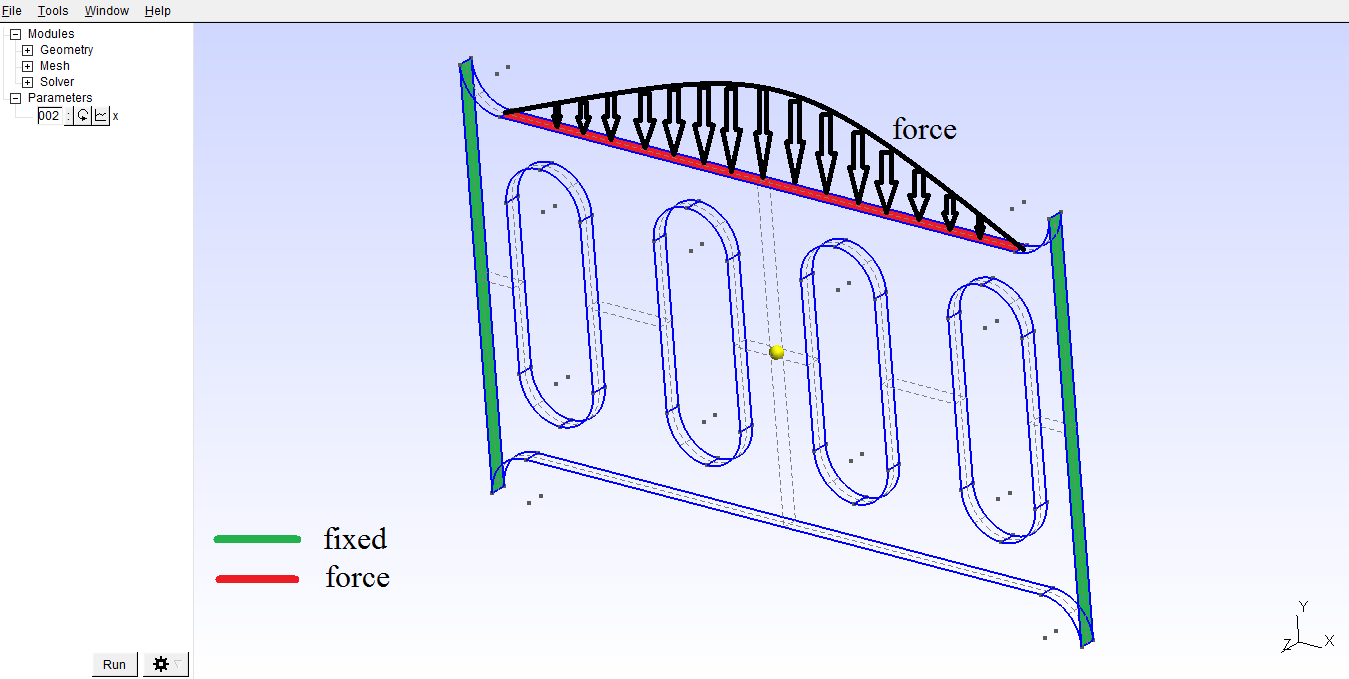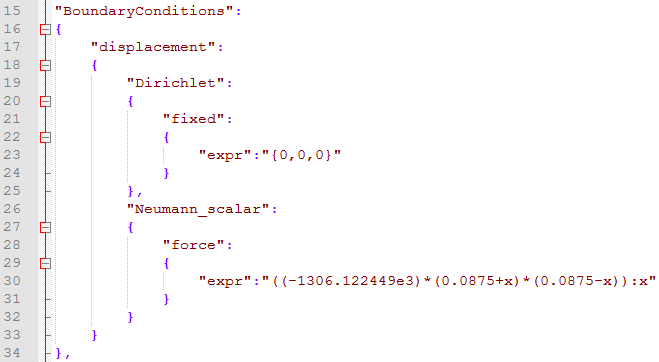## 5. Results

### 5.1. Displacement

Maximum displacement: 1.498e-07 m

 In the lower picture a scale factor was used to make the displacement easy to see. The distorted state is indicated by the wireframe model.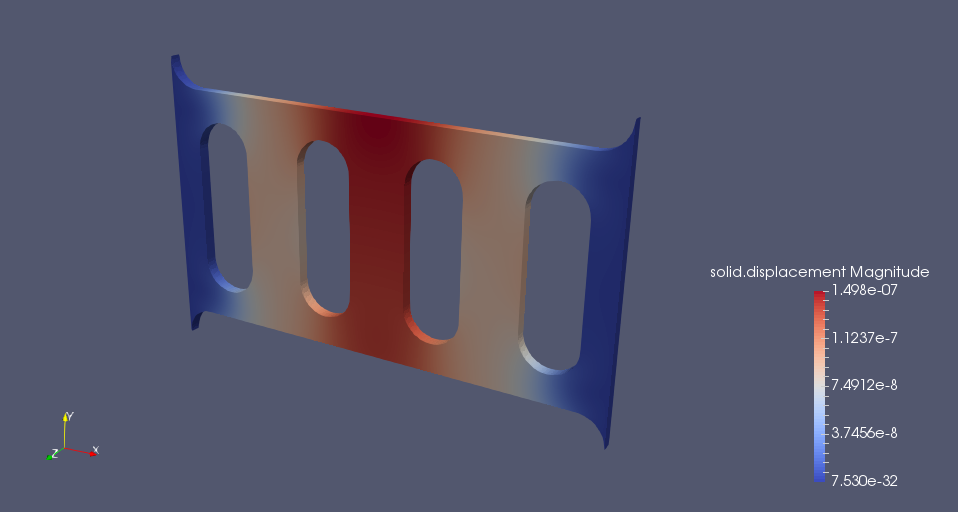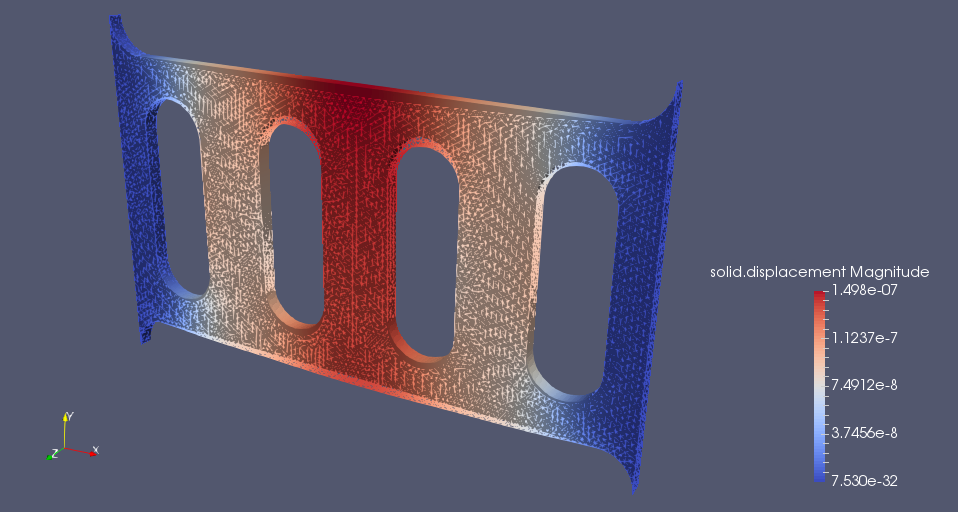### 5.2. Von-Mises Criterions

Maximum stress: 1.046e+05 Pa